In this module, I expand upon the Pure Locational Clustering (PLC) approach using various tools for defining and evaluating clusters. As we discussed in class, PLC refers to an approach where clusters are defined based on the geographic coordinates of a set of points (artifacts, sites, etc.) rather than any attributes associated with those points. In the example below, I will use the Barmose I dataset from your Ducke reading (2015; see also Blankholm 1991). Again, Barmose I is an early Maglemosian (ca. 7500-6000 B.C.) site in South Zealand, Denmark consisting of a hypothesized hut or sleeping area surrounded by a dense scatter of lithic artifacts. I will focus in particular on the K-means and DBSCAN methods but will also discuss the fuzzy K-means procedure. I’m leaving out the EM expectation maximization procedure but I will talk about that one a bit after you turn in this assignment.

For all clustering methods considered here there are a few decisions an analyst needs to make. For K-means/fuzzy K-means, the analyst much select the number of clusters to be returned. For the DBSCAN method, the analyst must determine the distance and number of points to be considered in calculating density to define clusters (DBSCAN - density based spatial clustering). In the example below I’ve provided some diagnostics that can help you make these decisions, but remember, these are merely heuristic approaches and shouldn’t be thought of as THE ONLY way to parameterize these clustering algorithms.

This module will rely primarily on the “cluster,” “spatstat,” “maptools,” and “dbscan” packages in R.

The first step is to read in the data and create a new object containing only the xy coordinates

``````# read in the Barmose data file, columns E=Easting, N=Northing, TYPE=artifact
# category, Label=artifact type name
# create new object using only the first two columns
Barmose.coord <- as.matrix(Barmose[, 1:2])``````

To get a sense of the spatial data, I can then plot the points with symbols indicating different artifact types (unlabeled for now)

``plot(Barmose.coord, pch = Barmose\$TYPE, cex = 0.5)``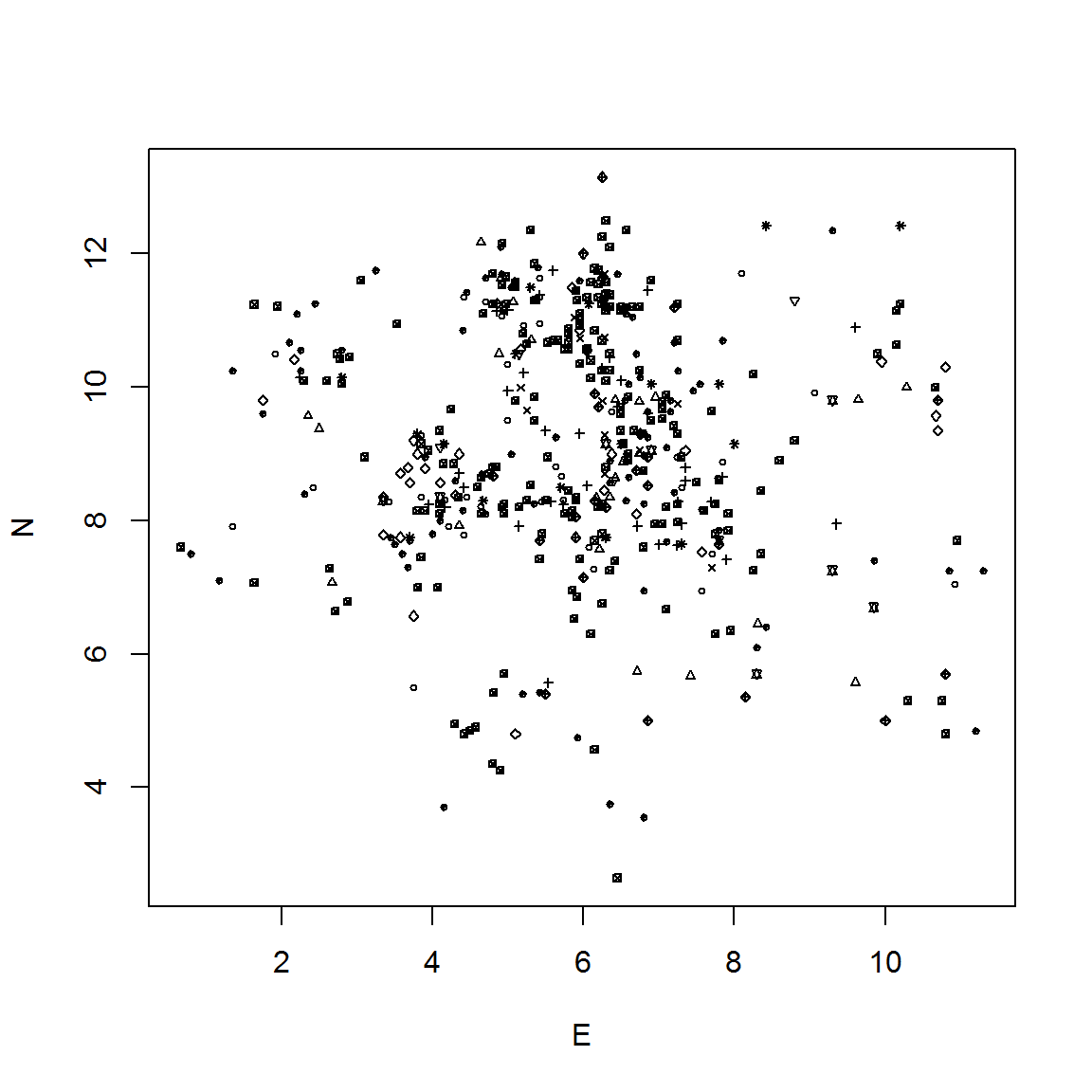## K-means clusters

We will first tackle the traditional K-means approach to defining clusters. K-means is a divisive non-hierarchical method of clustering. This is an iterative process, which means that at each step the membership of each individual in a cluster is reevaluated based on the current centers of each existing cluster. This is repeated until the desired number of clusters (or the number of individuals) is reached. Thus, it is non-hierarchical because an individual can be assigned to a cluster, and reassigned at any later stage in the analysis. Clusters are defined based on Euclidean distances so as to reduce the variability of individuals within a cluster, while maximizing the variability between clusters (known as the Sum of Squares Error or SSE).

One approach to choosing an appropriate number of clusters to test is to conduct a randomization procedure to assess changes in the global SSE for varying cluster levels in both the original data and in a large number of randomized versions of those data. In the code below, I create 1000 randomized versions of the points and then compare the SSE at each cluster solution to the actual data. The results are then plotted both in terms of absolute SSE and in terms of the difference between the actual and mean randomized data.The goal of these plots is to identify any inflection points in SSE values and/or the pattern of departures from random at varying cluster levels (see Kintigh 1990 for more details).

In the example below I will evaluate the first 15 cluster solutions.

``````# define function for calculating within group sum of squares for kmeans
# solutions 1 through max K
kmeans.wss <- function(data, maxk) {
wss <- (nrow(data) - 1) * sum(apply(data, 2, var))
for (i in 2:maxk) wss[i] <- sum(kmeans(data, centers = i, iter.max = 50, nstart = 10)\$withinss)
return(wss)
}

set.seed(1275)  #set random seed for repeatability
# calculate wss for cluster levels 1 through 15 for the original data
kmeans.orig <- kmeans.wss(Barmose.coord, maxk = 15)

# the following chunk creates 1000 column randomized version of the original
# dataset and calculates global SSE for cluster solutions 1 through 15. This will
# take some time to calculate
rand.out <- NULL
nsim <- 1000
for (i in 1:nsim) {
rand.tab <- apply(Barmose.coord, 2, sample)
rand.out <- rbind(rand.out, kmeans.wss(rand.tab, maxk = 15))
}

# next I plot the SSE scores by cluster solution for the original and the mean of
# the randomized data
plot(log(kmeans.orig), type = "b", col = "blue", xlab = "Number of Clusters", ylab = "Sum of Squares")
points(log(colMeans(rand.out)), type = "l", col = "red", lty = 2)
legend("topright", c("Original Data", "Mean of 1000 Random Runs"), col = c("blue",
"red"), lty = c(1, 2))``````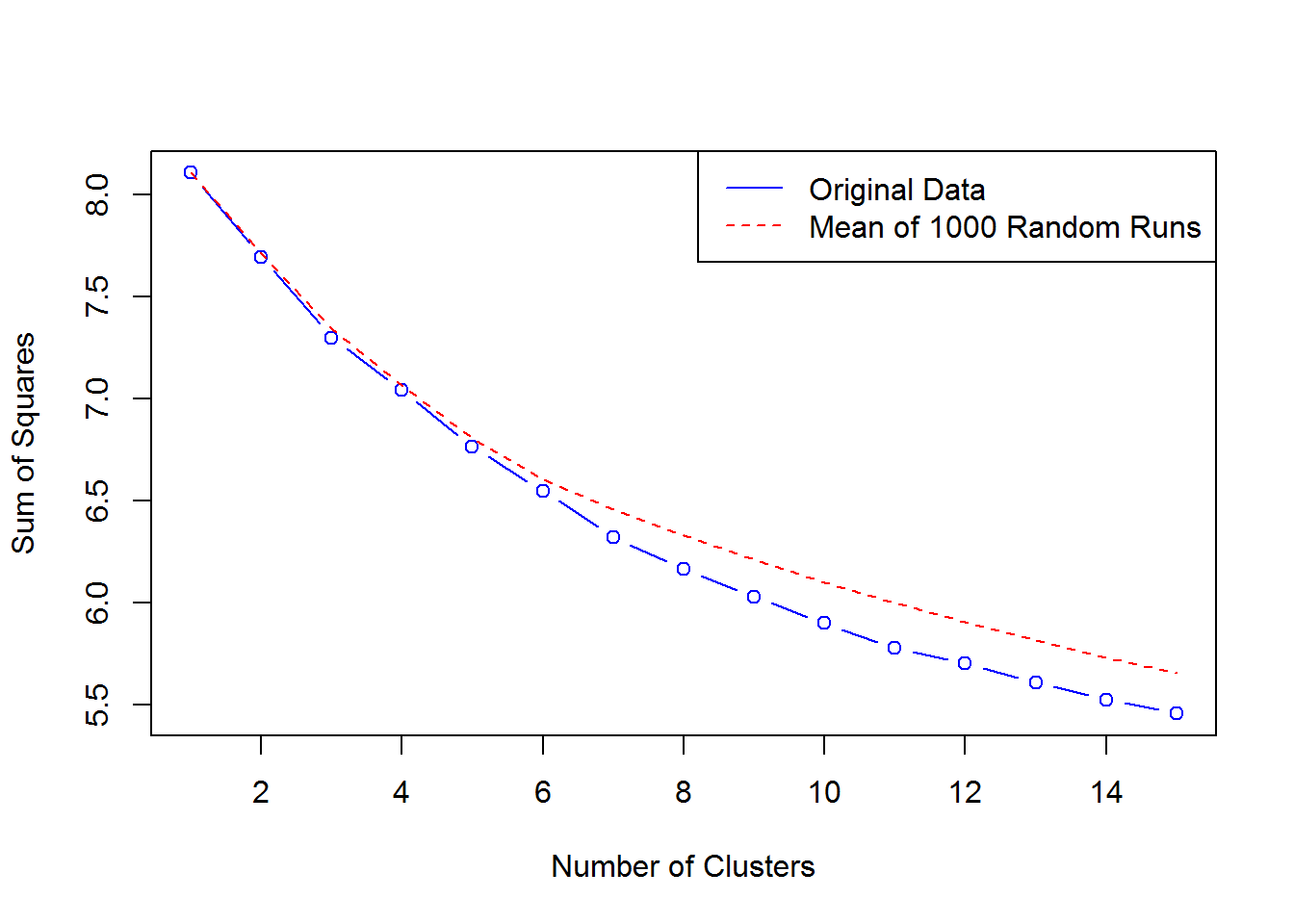``````# finally, I plot the difference between the mean random SSE and the actual SSE
# from the original data
plot(log(colMeans(rand.out)) - log(kmeans.orig), type = "b", col = "blue", xlab = "Number of Clusters",
ylab = "Actual SSE - Mean Randomized SSE")``````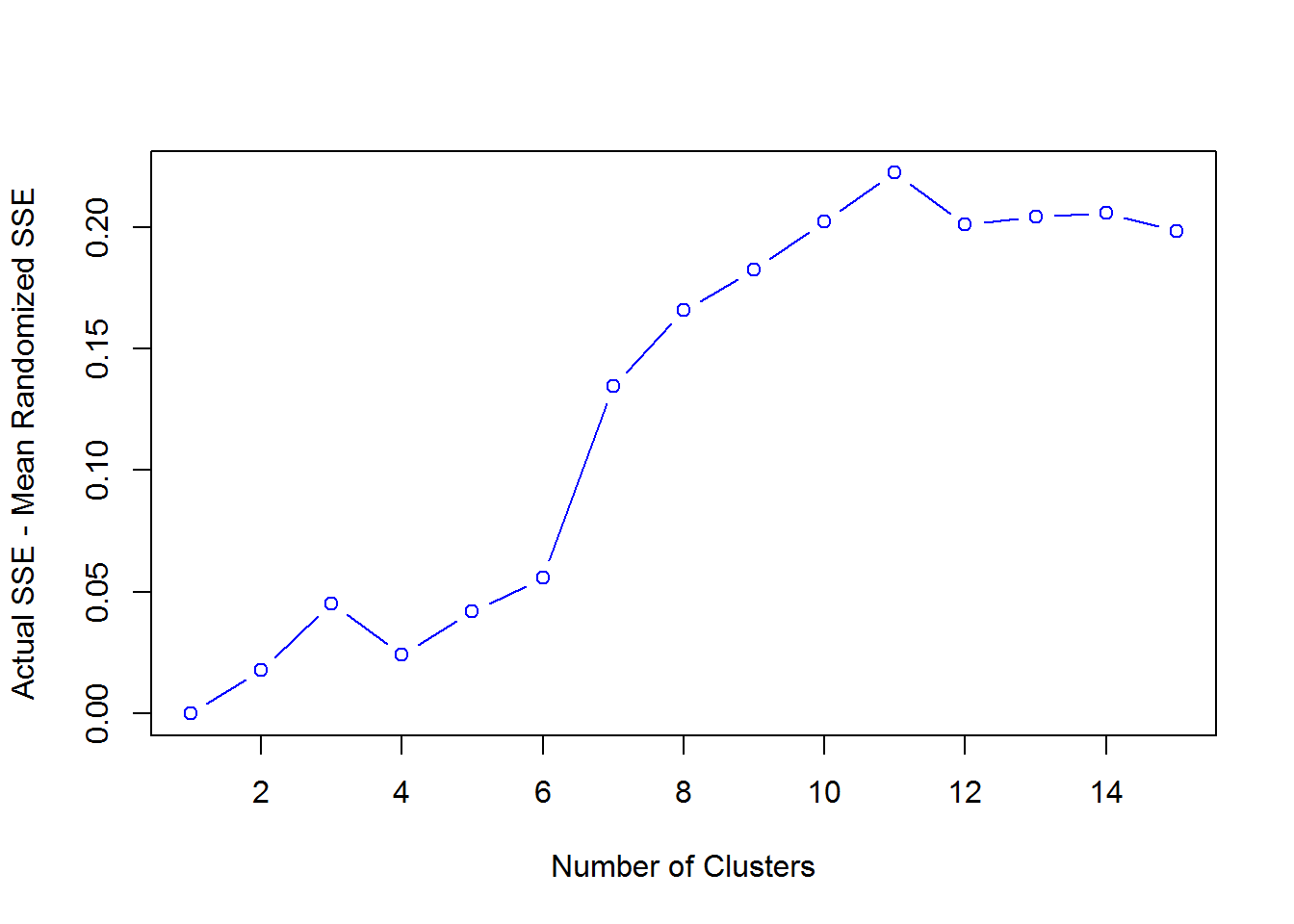Note in the plots above the actual SSE declines faster than the mean randomized SSE at increasing cluster solutions. It appears here that the difference between actual and randomized data is greatest at the 13 cluster solution. There is also a big difference between the 6 cluster solution and the 7 cluster solution and only small difference thereafter. For the purposes of this example, I select the 8 cluster solution for further evaluation as this is about the point where differences between random and actual SSE apear to level off. It seems that the other cluster solutions may also be worth exploring in the future, though I don’t do that here. Remember, it is good practice to test different solutions and evaluate their impact on the results. Note that the 6 cluster solution was selected by Ducke based on externally derived expectations.

In the code below I calculate the 8 cluster solution and then plot the results on the map, color coded by cluster.

``````# calculate the 8 cluster soultion and extract cluster assignments
fitK <- kmeans(Barmose.coord, 8, iter.max = 100, nstart = 100)\$cluster
# plot the results
plot(Barmose.coord, pch = Barmose\$TYPE, cex = 0.5, col = fitK)
legend("bottomleft", as.character(seq(1:8)), pch = 16, col = seq(1:8))``````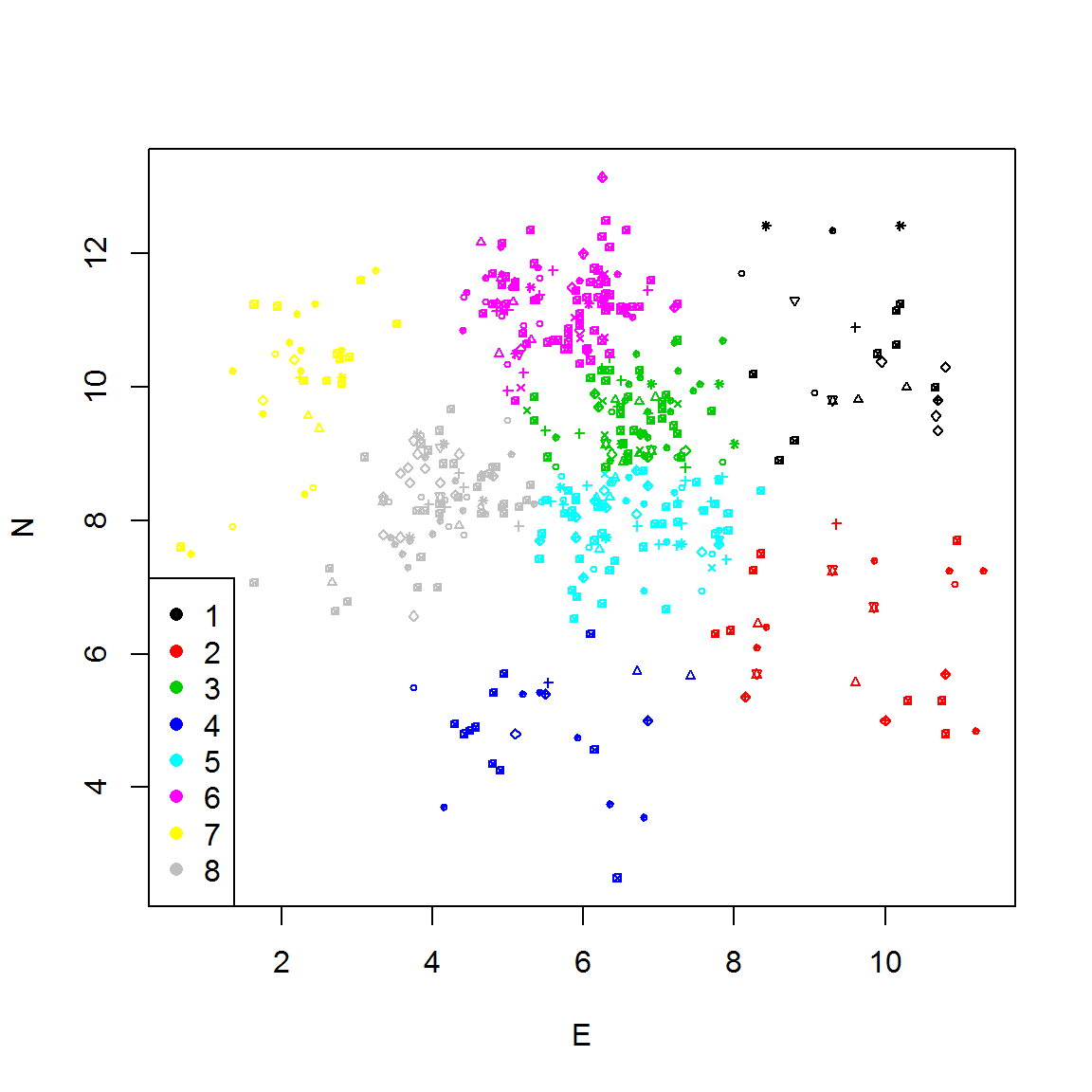As this image shows there are several dense clusters in the central portion of the site and a few sparser clusters around the edges. Since density differs pretty dramatically across the site as a whole, it might be worth considering a density based clustering algorithm.

## DBSCAN - Density Based Clustering

The DBSCAN clustering algorithm is a density based algorithm that groups points based on their relative to user provided parameters. DBSCAN creates neighborhoods around core points of a specified diameter. Core points are defined as those points where a specified minimum number of points falls within the specified diameter from that point. The algorithm identifies all cores points and then determines which core points are mutually reachable. These are then connected until no more points can be added. All of these connected core points and all the non-core points within their neighborhoods are then defined as a single cluster (and multiple clusters are defined in the same way across a sampled area). All non-core points that are not reachable by any core point are excluded and considered “noise” and not explicitly assigned to a cluster.

Thus, for this clustering algorithm we do not choose how many clusters to define as this is generated by the algorithm itself. Instead, we must select parameters regarding the distances across which the density neighborhoods will be evaluated and the minimum number of points to include in a neighborhood. There are a few diagnostics that we can use to help us parameterize this algorithm including kernal density maps and variations on Ripley’s K.

The first thing we need to is to convert the Barmose dataset into a ppp Point Pattern object as required by the ‘spatstat’ package. We will also import a shapefile defining the limits of excavation at Barmose because, as we previously discussed, Ripley’s K is sensitive to the shape and size of the boundary area selected (see Ripley’s K module for more details). For good measure we’ll plot the resulting excavation limits and points in this new ppp format with artifacts symbolized by type.

``````# Initialize required libraries
library(spatstat)
library(maptools)

# Import shapefile for grid and covert to SpatialPolygons object
Excav <- as(Excav, "SpatialPolygons")
# this line dfines the window to be used in Ripley's K calculations
W <- as(Excav, "owin")

# Define ppp object based on Barmose point locations
Barmose.pp <- ppp(x = Barmose\$E, y = Barmose\$N, window = W, marks = Barmose\$Label)

plot(Barmose.pp, cex = 0.5)``````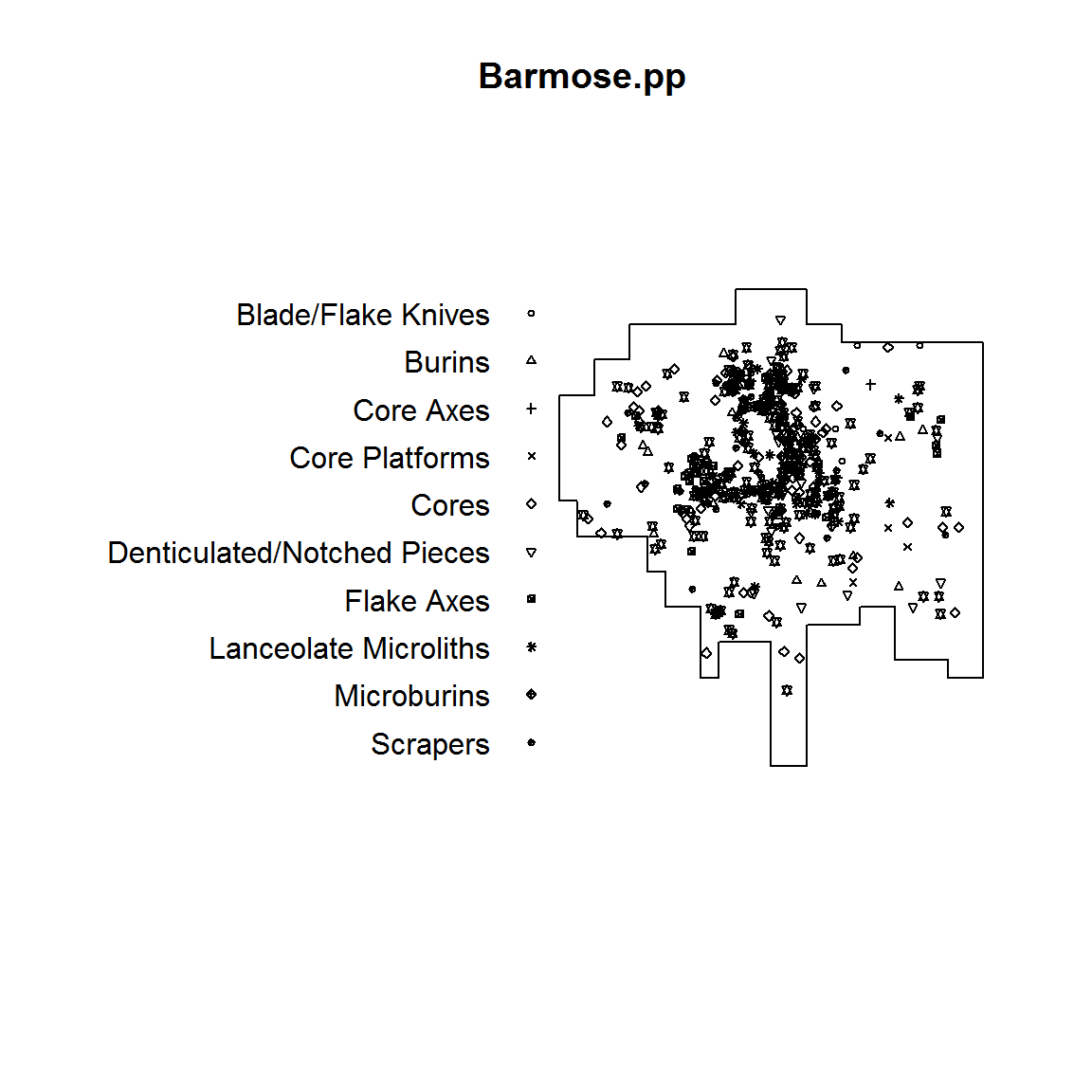Next, I create a series of kernal density maps of the point locations across the Barmose site. For these maps I change the sigma scaling parameter to explore structure at the site at varying distances. For this example, I explore values between 0.1 and 0.6 at intervals of 0.1.

``````par(mfrow = c(2, 3))  # set up plot for two rows and three columns
# plot density maps at various sigma values
plot(density(Barmose.pp, sigma = 0.1))
plot(density(Barmose.pp, sigma = 0.2))
plot(density(Barmose.pp, sigma = 0.3))
plot(density(Barmose.pp, sigma = 0.4))
plot(density(Barmose.pp, sigma = 0.5))
plot(density(Barmose.pp, sigma = 0.6))``````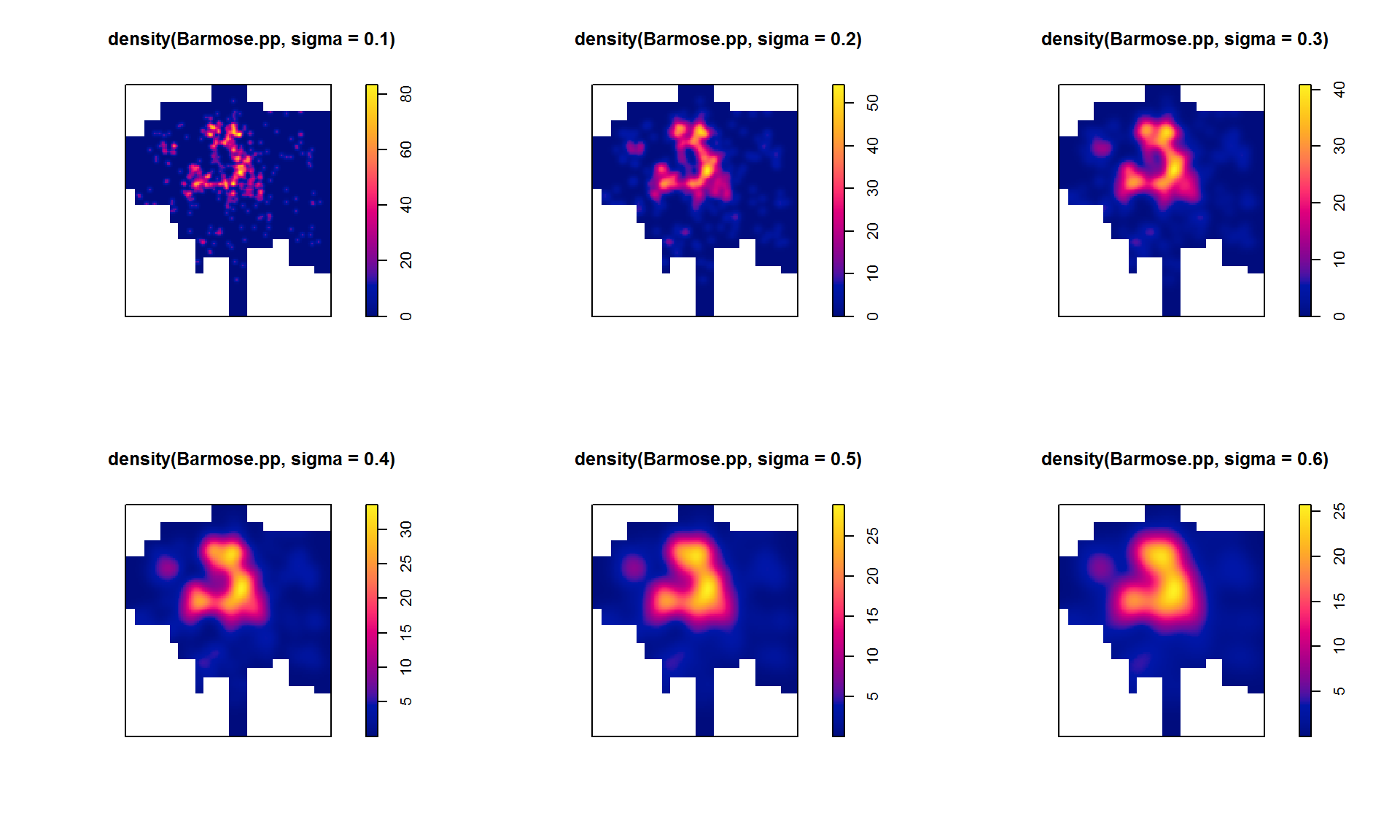As these density plots show, a sigma value of 0.1 shows many very small pockets of density while values of 0.4 through 0.6 essentially smear the point distribution into a single blob. Intermediate values at about 0.2 and 0.3 seem to represent a decent compromise.

Before I select a distance, however, I want to evaluate clustering using the Ripley’s K approach. For all of the examples below I’m going to use the variance adjusted version of Ripley’s K defined as L-hat. I first calculate L-hat for the entire dataset and then plot those values across a range of plausible distances in relation to both the theoretical expectation and the envelope of values defined based on 99 simulations of complete spatial randomness within the area bounded by the limit of excavation (see Ripley’s K overview). I then replot the same data with L centered at 0 for all distances to look at deviations between the observed and theoretical expectation at different distances. I use the Ripley correction for edge effects here. This calculation may take several seconds.

``````par(mfrow = c(1, 2))  #set up for two plots on one row
# calculate L-hat within windown 'W' using the Ripley correction
L <- envelope(Barmose.pp[W], Lest, correction = "Ripley", verbose = F)

# plot L-hat against distance
plot(L, xlab = "Meters", ylab = expression(hat("L")))
# plot L-hat recentered at 0
plot(L, . - r ~ r, xlab = "Meters", ylab = expression(hat("L")), main = "L recentered")``````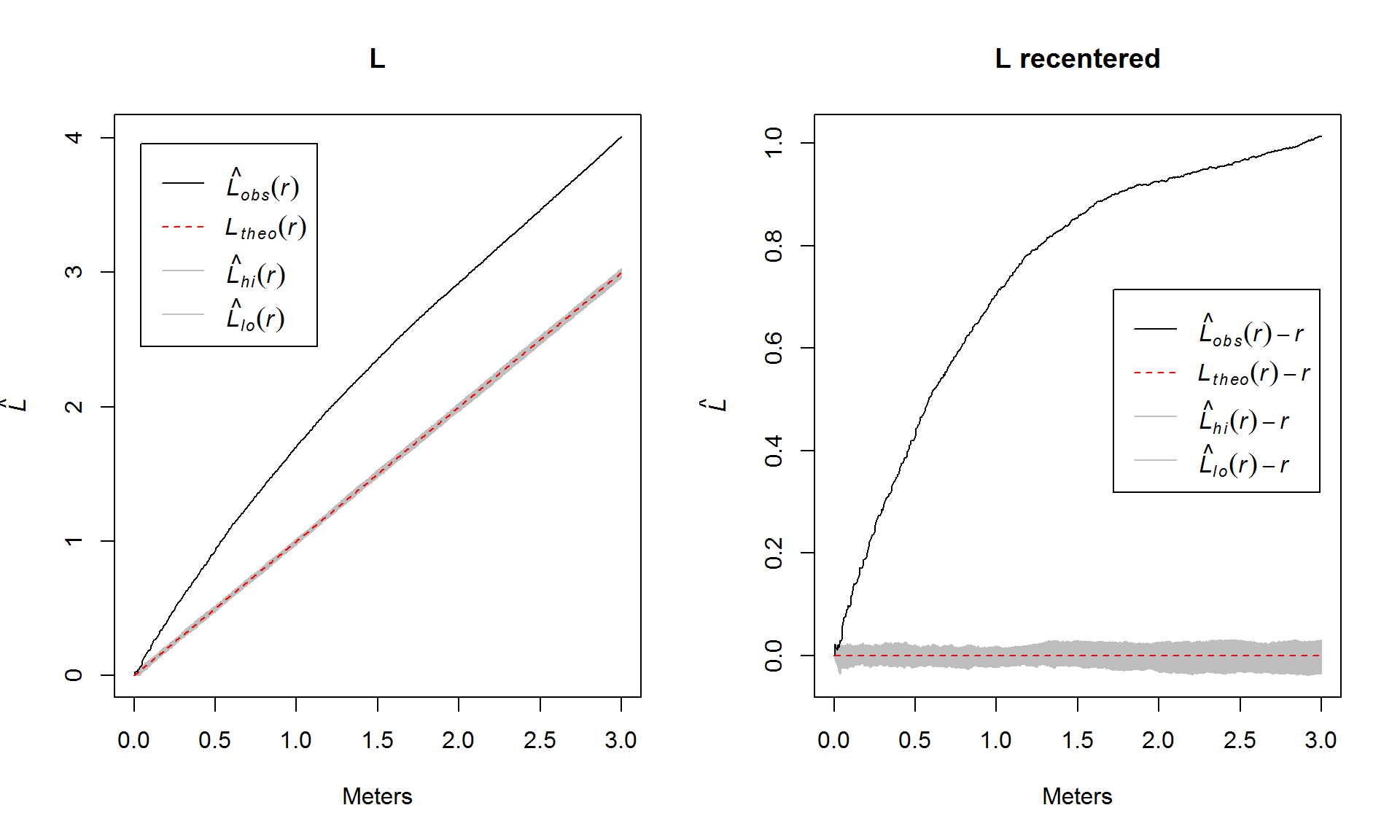As these plots indicate, the Barmose point locations are considerably more clustered than would be expected for complete spatial randomness at essentially all distances but the rate of increase in the difference between the observed and simulated points starts to level off after 1.5 meters or so. The next question I might ask is how this pattern plays out for particular artifact types. To explore this, I plot re-centered L-hat plots for several artifact categories (those with at least 15 objects) to compare the results. This time for the sake of calculation time I’ll simply plot the results against the theoretical expectation and forego the simulations (but it would be a good idea to check that later).

``````par(mfrow = c(3, 3))  # set up for plotting
# set up vector of artifact type names and remove categories with fewer than 15
# artifacts
B.lab <- levels(Barmose.pp\$marks)[-c(1, 3, 4)]

# plot recentered L-hat plot for each artifact type
for (i in 1:length(B.lab)) {
plot(Lest(Barmose.pp[Barmose.pp\$marks == B.lab[i]], correction = "Ripley", rmax = 1.5),
. - r ~ r, xlab = "Meters", ylab = expression(hat("L")), main = B.lab[i])
}``````